# Plenary lectures

### Saturday January 23

9:00-10:00
Marta Sanz-Solé (Universitat de Barcelona)
Hitting probabilities for solutions to stochastic partial differential equations

We will present some results on upper and lower bounds for hitting probabilities of random fields in terms of Hausdorff measure and Bessel-Riesz capacity, respectively. Applications to several examples of stochastic partial differential equations will be discussed.

Alternating sign matrices are one of those fascinating combinatorial objects that admit an exceptional simple enumeration formula while at the same time proving this formula is rather complicated. They were first defined and studied in the early 1980s by Robbins and Rumsey in connection with Dodgson's condensation method for computing determinants. The research was further stimulated after the discovery of the relation to a statistical mechanics model (six-vertex model) for square ice''. In the talk I will first give an introduction into this field and then present our approach to refined enumerations of alternating sign matrices.

Classical Bessel potential spaces, introduced by N. Aronszajn, K. Smith and A. P. Calderón in 1961, have played a significant role in mathematical analysis and in applications for many years. These spaces are modelled upon the scale of Lebesgue spacesand they coincide with the Sobolev spaceswhen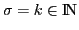and. However, it has gradually become clear that to handle some situations (especially limiting ones) a more refined tuning is desirable.

For this purpose, we replace the Lebesgue spacesby rearrangement invariant Banach function spaces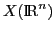and we study embeddings of Bessel potential spaceswith order of smoothness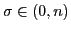into generalized Hölder spaces defined by means of the k-modulus of smoothness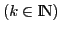. We establish necessary and sufficient conditions for such embeddings and we present applications of our results. In particular, we obtain new and sharp embeddings of Sobolev-Orlicz spacesand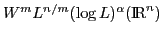into generalized Hölder spaces, which improve known results.

The lecture is based on a joint work with Amiran Gogatishvili and Júlio S. Neves.

We give an overview of Thurston's geometrization conjecture, the Ricci flow introduced by Hamilton, and Perelman's proof.

It is well known that one can test in linear time whether a given graph is planar. We consider the higher-dimensional generalization of this problem: given a k-dimensional simplicial complex K and a target dimension d, does K embed into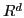? Surprisingly, rather little seems to be known about the algorithmic aspects of this problem. (All the relevant topological notions will be defined and explained during the talk, at least on an intuitive level.) Known results easily imply that the problem is solvable in polynomial time if k=d=2 or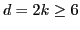. We show that the problem is algorithmically undecidable for k=d-1 and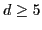. This follows from a famous result of Novikov on the unsolvability of recognizing the 5-sphere. Our main result is NP-hardness in the rangeand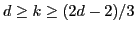. These dimensions fall outside the so-called metastable range of a theorem of Haefliger and Weber, which characterizes embeddability in terms of the so-called deleted product obstruction. Our reductions are based on examples, due to Segal, Spiez, Freeman, Krushkal, Teichner, and Skopenkov, showing that outside the metastable range, the deleted product obstruction is insufficient. Joint work with Martin Tancer and Uli Wagner.

A survey of recent mathematical results on quantum gases in fast rotation and the appearance and disappearance of vortices will be presented.

### Sunday January 24

15:00-16:00
Fernando Muro (University of Seville)
Representability of cohomology theories

Brown and Adams representability theorems for cohomology theories originated in algebraic topology. They have been extended to other algebraic and geometric contexts, where they have been successfully applied (Grothendieck duality in algebraic geometry, Auslander-Reiten theory in representation theory, motivic cohomology?). Nevertheless, there are still many open questions with potentially striking applications. We will introduce the audience to what is known and report on recent advances.

Discrete dynamical systems given by a continuous map on a topological (usually compact metrizable) space will be considered. Minimality of such a system/map can be defined as the density of all forward orbits. Every compact system contains at least one minimal set, i.e., a nonempty closed invariant subset such that the restriction of the map to this subset is minimal. A fundamental question in topological dynamics is the one on the topological structure of minimal sets of continuous maps in a given space (and, in particular, whether the space itself admits a minimal map or not).

We will present some known as well as new results on topology of minimal sets, some tools used in the proofs, and some open problems.

Graph covers emerged forty years ago in the context of maps on surfaces, appropriately adapted to reflect the discrete nature of these objects;

the primary incentive was the final solution of the long standing Heawood's Map Colour Problem.

Much around the same time these techniques became an indispensable tool in algebraic graph theory; here, the motivation came from problems related to classification of graphs and maps in terms of their symmetries. An important topic in this setting is the problem of lifting automorphisms, a problem that has long been well understood at a very general level in algebraic topology. These general results, however, are rather inadequate if we are to successfully cope with concrete examples and specific questions that arise when studying symmetry from a combinatorial point of view in the setting of graphs and maps. A number of papers dealing with the lifting problem have been published in the past fifteen years. In terms of content they range from general considerations to more concrete applications in the field of graphs and maps on surfaces, dealing with enumeration, classification and construction of infinite families of graphs with specific symmetry properties, and compiling lists of such graphs up to a certain order.

In the talk I will review some results covering this topic.